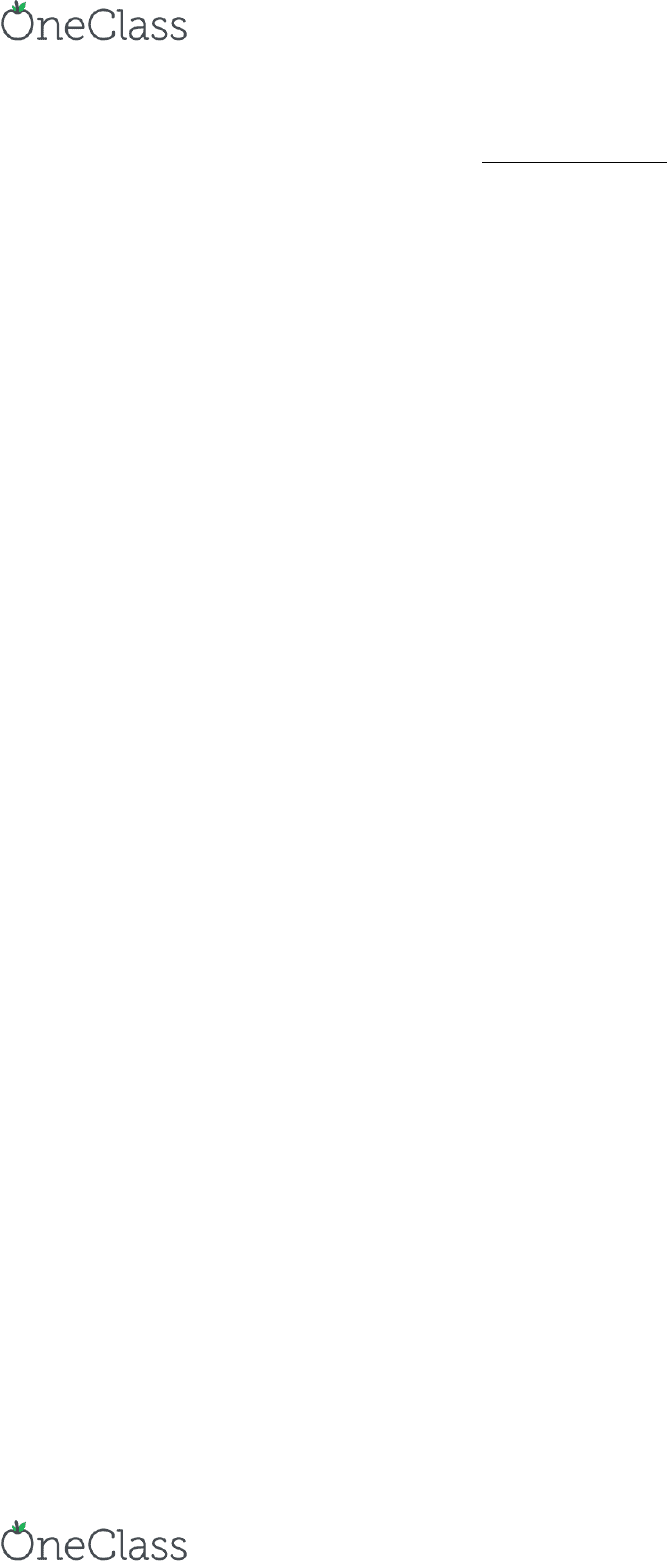Textbook Notes (290,000)
US (110,000)
UCSC (100)
AMS (30)
AMS 5 (20)
Chapter 9

# AMS 5 Chapter Notes - Chapter 9: Hiram Johnson, Longitudinal Study, Dependent And Independent Variables

Department
Applied Math and Statistics
Course Code
AMS 5
Professor
Yonatan Katznelson
Chapter
9

This preview shows half of the first page. to view the full 3 pages of the document.AMS 5 Practice 7
4/16/2017
(8:00-9:05)
Chapter 9, #2, 3, 4, 7, 8, 10, 11
2. True or false, and explain briefly:
(a) If the correlation coefficient is -0.80, below-average values of the
dependent variable are associated with below-average values of the
independent variable.
(b) If y is usually less than x, the correlation coefficient between x and y will
be negative.
2a) True; the negative simply means a correlation with a negative slope, not that there isn’t a
correlation at all.
2b) False; x > y gets you a positive sloped line, which also gives you a positive correlation
coefficient.
3. In each case, say which correlation is higher, and explain briefly. (Data is from a
longitudinal study of growth.)
(a) Height at age 4 and height at age 18, height at age 16 and height at age 18.
(b) Height at age 4 and height at age 18, weight at age 4 and weight at age 18.
(c) Height and weight at age 4, height and weight at age 18.
3a) Second case, since the difference between a 16 year old and an 18 year old aren’t that
profound.
3b) Equal, since profound changes occur between the age groups and affect both age and
weight.
3c) First case, since there is a wider range of heights/weights when you’re older versus age 4.
4. An investigator collected data on heights and weights of college students; results can be
summarized as follows.
find more resources at oneclass.com
find more resources at oneclass.com